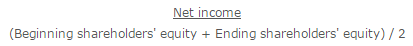# What is Return on Average Equity?

The return on average equity measures the performance of a company based on its average shareholders’ equity outstanding. The measure is especially useful in situations where a business has been actively selling or buying back its shares, issuing large dividends, or experiencing significant gains or losses.

The basic return on equity formula is simply net income divided by shareholders’ equity. However, the denominator in this formula is based on the ending shareholders’ equity figure in the balance sheet, which could include last-minute stock sales,  repurchases, dividend payments, and so forth. The result may be a return on equity figure that does not accurately reflect the actual return over the entire measurement period.

A better approach is to develop an average equity figure. The simplest approach is to add together the beginning and ending shareholders’ equity figures and divide by two. However, if there were a number of equity transactions during the period to which the net income figure applies, it may be necessary to develop a more refined average. For example, it might be an average that includes the ending shareholders’ equity figure for each month of the year, which is then divided into the net income figure for the entire year. The result is a more accurate measurement outcome.

Based on this discussion, the formula for the return on average equity is:For example, a business earns \$100,000 in annual net income. Its beginning shareholders’ equity was \$750,000 and its ending shareholders’ equity is \$1,000,000. The calculation of its return on average equity is:If a business rarely experiences significant changes in its shareholders’ equity, it is probably not necessary to use an average equity figure in the denominator of the calculation.